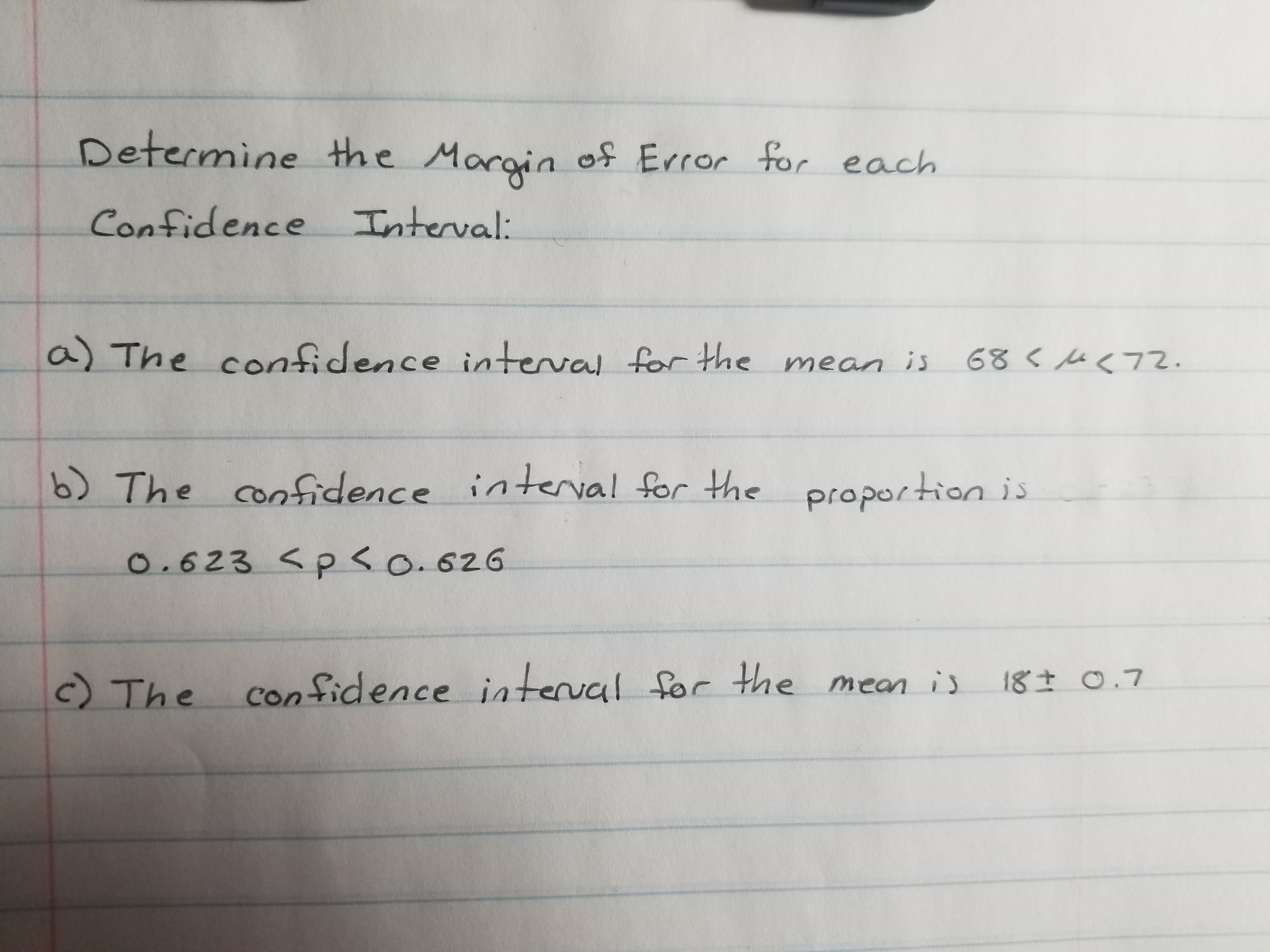# Determine the Margin of Error for eachConfidence Interval:a) The confidence inteval forthe mean is6872.6) The confidence inteval for the proportiono.623 p o.626c TheConfidence iateval for the mean is18t O.7

Question
71 views

I cant find an example for how to solve this. Help would be so great! Thank youhelp_outlineImage TranscriptioncloseDetermine the Margin of Error for each Confidence Interval: a) The confidence inteval forthe mean is 68 72. 6) The confidence inteval for the proportion o.623 p o.626 c The Confidence iateval for the mean is 18t O.7 fullscreen
check_circle

star
star
star
star
star
1 Rating
Step 1

Margin of error:

The formula of margin of error for confidence interval is as follows:

Step 2

a) Computation of margin of error for the confidence interval (68<µ<72):

The confidence interval for the mean is given as 68<µ<72.

Here, the upper bound is 72 and the lower bound is 68.

The margin of error for the confidence interval (68<µ<72) is obtained as 2, from the calculation given below:

Step 3

b) Computation of margin of error for the confidence interval (0.623<p<0.626):

The confidence interval for the proportion is given as 0.623<p<0.626.

Here, the upper bound is 0.626 and the lower bou...

### Want to see the full answer?

See Solution

#### Want to see this answer and more?

Solutions are written by subject experts who are available 24/7. Questions are typically answered within 1 hour.*

See Solution
*Response times may vary by subject and question.
Tagged in

### Other